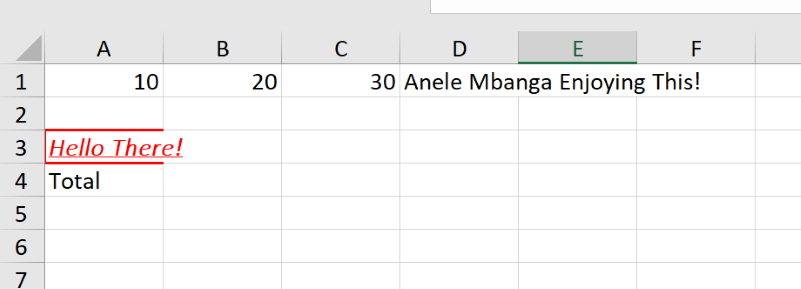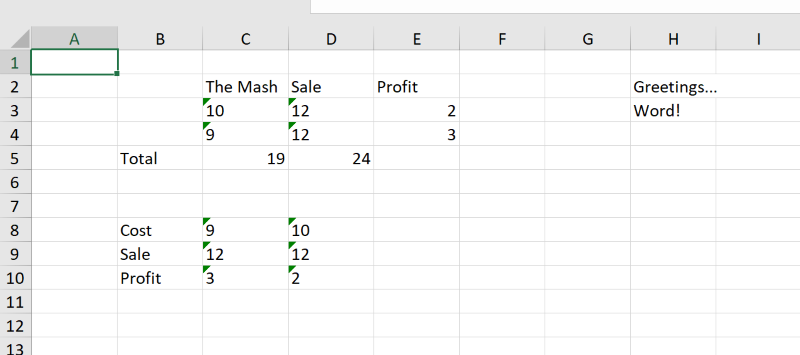# B4J Library[BANanoOXML] Client Side Excel Report Generation

Yippie!!

In case you need something like this one day, so here is my BANano implementation of it for y'all. With this one is able to CREATE Excel workbooks with multiple sheets. One is able to also set styles e.g. bold, italic, forecolor, add borders. Once generated, the workbook is automatically downloaded. So happy to have found this lib on the net.Usage:

In AppStart

B4X:
``````BANano.Header.AddJavascriptFile("fileSaver.min.js")

B4X:
``````Private oxml As BANanoOXML
oxml.Excel("themash.xlsx")
Dim wkSheet As BANanoObject = oxml.WorkSheet("TheMash")
oxml.SetNumber(wkSheet, 1, 1 , 10)
oxml.SetNumber(wkSheet, 1, 2, 20)
oxml.SetNumber(wkSheet, 1, 3, 30)
'
oxml.SetText(wkSheet, 3,1, "Hello There!")
'
oxml.SetSharedText(wkSheet, 4, 1, "Total")
'
oxml.SetFormula(wkSheet,1,4, "SUM(A1:C1)")``````

You can set styles on cells like this...

B4X:
``````Dim cellx As BANanoObject = oxml.GetCell(wkSheet, 3, 1)
Dim style As OXMLStyle = oxml.CreateStyle
style.bold = True
style.fontColor = "ff0000"
style.italic = True
style.underline = True
style.fontName = "Calibri Light"
style.borderColor = "ff0000"
style.borderThickness = oxml.BorderThick
oxml.SetStyle(cellx, style)

'get cell
Dim cell As BANanoObject = oxml.GetCell(wkSheet, 1, 4)
oxml.SetCellText(cell, "Anele Mbanga Enjoying This!")``````

B4X:
``oxml.download(Me, "onDownload")``

B4X:
``````Sub onDownload
End Sub``````

Have Fun!

#### Attachments

• BANanoOXML.zip
46.3 KB · Views: 252
Last edited:

#### Mashiane

##### Expert
Longtime User
I also tested adding another worksheet on the file, worked like a charm.

B4X:
``````'create another sheet
Dim sheet1 As BANanoObject = oxml.WorkSheet("Sheet1")
'create row data
oxml.SetRow(sheet1, 2, 3, Array("Cost", "Sale", "Profit"))
oxml.SetRow(sheet1, 3, 3, Array("10", "12"))
oxml.SetRow(sheet1, 4, 3, Array("9", "12"))
'
oxml.SetFormula1(sheet1, 3, 5, "(D3 - C3)", "2")
oxml.SetFormula1(sheet1, 4, 5, "(D4 - C4)", "3")
'
Dim r5c2 As OXMLRow = oxml.CreateRow(5, 2)
oxml.SetRow1(sheet1, r5c2)
'
'set column values
oxml.SetColumn(sheet1, 8, 2, Array("Cost", "Sale", "Profit"))
oxml.SetColumn(sheet1, 8, 3, Array("9", "12", "3"))
oxml.SetColumn(sheet1, 8, 4, Array("10", "12", "2"))

'update column
oxml.SetColumn(sheet1, 2, 8, Array("Hello", "Word!"))
Dim col As BANanoObject = oxml.GetColumn(sheet1, 2, 8)
oxml.SetColBO(col, Array("Greetings..."))

'get an existing row
Dim row As BANanoObject = oxml.GetRow(sheet1, 2, 3)
oxml.SetRowBO(row, Array("The Mash"))``````

The result is..•swamisantosh and joulongleu

#### Mashiane

##### Expert
Longtime User
Update

1. Merging Cells
2. Applying fills
3. Horizontal & Vertical Alignment

The creator of this library was kind enough to honour my request for additional features here.To merge the cells, we have run:

B4X:
``````'merge cells
oxml.Merge(wkSheet, "D2:F5")``````

We have applied horizontal and vertical alignment on B1.

B4X:
``````'get a cell and format alignment
'Possible values for vAlignment are top, center, bottom, and that of hAlignment are left, center, and bottom.
Dim r1c2 As BANanoObject = oxml.GetCell(wkSheet, 1, 2)
Dim cs As OXMLStyle = oxml.CreateStyle
cs.vAlignment = "center"
cs.hAlignment = "center"
cs.bold = True
oxml.SetStyle(r1c2, cs)``````

On B2, we have applied some number formatting:

B4X:
``````'Some of the standard defined numeric formats are "mm-dd-yy";
'"m/d/yy h:mm"; "#,##0 ;(#,##0)"; "#,##0 ;[Red](#,##0)"; "#,##0.00;(#,##0.00)"; "#,##0.00;[Red](#,##0.00)";
'"mmss.0"; "yyyy-mm-dd". For more number format expressions, refer open xml documentation.
Dim r2c2 As BANanoObject = oxml.GetCell(wkSheet, 2, 2)
oxml.SetCellNumber(r2c2, -4123.89)
Dim cs As OXMLStyle = oxml.CreateStyle
cs.numberFrmat = "#,##0.00 ;[Red](#,##0.00)"
oxml.SetStyle(r2c2, cs)``````

On A10, we have set up a fill.

B4X:
``````'try and set a border
Dim r2c4 As BANanoObject = oxml.GetCell(wkSheet, 10, 1)
oxml.SetCellNumber(r2c4, 1000.00)
Dim cs As OXMLStyle = oxml.CreateStyle
cs.FillPattern = oxml.pattern_solid
cs.FillForeColor = oxml.colors_yellow
cs.FillBackground = oxml.colors_white
oxml.SetStyle(r2c4, cs)``````

#Source Code in first post

#### Mashiane

##### Expert
Longtime User
The complete source code for this...

B4X:
``````Sub ExcelReport
Private oxml As BANanoOXML
oxml.Excel("themash.xlsx")
Dim wkSheet As BANanoObject = oxml.WorkSheet("TheMash")
oxml.SetNumber(wkSheet, 1, 1 , 10)
oxml.SetNumber(wkSheet, 1, 2, 20)
oxml.SetNumber(wkSheet, 1, 3, 30)
'
oxml.SetText(wkSheet, 3,1, "Hello There!")
'
oxml.SetSharedText(wkSheet, 4, 1, "Total")
'
oxml.SetFormula(wkSheet,1,4, "SUM(A1:C1)")
'
Dim cellx As BANanoObject = oxml.GetCell(wkSheet, 3, 1)
Dim style As OXMLStyle = oxml.CreateStyle
style.bold = True
style.fontColor = "ff0000"
style.italic = True
style.underline = True
style.fontName = "Calibri Light"
style.borderColor = "ff0000"
style.borderThickness = oxml.BorderDouble
oxml.SetStyle(cellx, style)

'get cell
Dim cell As BANanoObject = oxml.GetCell(wkSheet, 1, 4)
oxml.SetCellText(cell, "Anele Mbanga Enjoying This!")
'
'get a cell and format alignment
'Possible values for vAlignment are top, center, bottom, and that of hAlignment are left, center, and bottom.
Dim r1c2 As BANanoObject = oxml.GetCell(wkSheet, 1, 2)
Dim cs As OXMLStyle = oxml.CreateStyle
cs.vAlignment = "center"
cs.hAlignment = "center"
cs.bold = True
oxml.SetStyle(r1c2, cs)

'Some of the standard defined numeric formats are "mm-dd-yy";
'"m/d/yy h:mm"; "#,##0 ;(#,##0)"; "#,##0 ;[Red](#,##0)"; "#,##0.00;(#,##0.00)"; "#,##0.00;[Red](#,##0.00)";
'"mmss.0"; "yyyy-mm-dd". For more number format expressions, refer open xml documentation.
Dim r2c2 As BANanoObject = oxml.GetCell(wkSheet, 2, 2)
oxml.SetCellNumber(r2c2, -4123.89)
Dim cs As OXMLStyle = oxml.CreateStyle
cs.numberFrmat = "#,##0.00 ;[Red](#,##0.00)"
oxml.SetStyle(r2c2, cs)

'merge cells
oxml.Merge(wkSheet, "D2:F5")
'
'try and set a border
Dim r2c4 As BANanoObject = oxml.GetCell(wkSheet, 10, 1)
oxml.SetCellNumber(r2c4, 1000.00)
Dim cs As OXMLStyle = oxml.CreateStyle
cs.FillPattern = oxml.pattern_solid
cs.FillForeColor = oxml.colors_yellow
cs.FillBackground = oxml.colors_white
oxml.SetStyle(r2c4, cs)

'
'create another sheet
Dim sheet1 As BANanoObject = oxml.WorkSheet("Sheet1")
'create row data
oxml.SetRow(sheet1, 2, 3, Array("Cost", "Sale", "Profit"))
oxml.SetRow(sheet1, 3, 3, Array("10", "12"))
oxml.SetRow(sheet1, 4, 3, Array("9", "12"))
'
oxml.SetFormula1(sheet1, 3, 5, "(D3 - C3)", "2")
oxml.SetFormula1(sheet1, 4, 5, "(D4 - C4)", "3")
'
Dim r5c2 As OXMLRow = oxml.CreateRow(5, 2)
oxml.SetRow1(sheet1, r5c2)
'
'set column values
oxml.SetColumn(sheet1, 8, 2, Array("Cost", "Sale", "Profit"))
oxml.SetColumn(sheet1, 8, 3, Array("9", "12", "3"))
oxml.SetColumn(sheet1, 8, 4, Array("10", "12", "2"))

'update column
oxml.SetColumn(sheet1, 2, 8, Array("Hello", "Word!"))
Dim col As BANanoObject = oxml.GetColumn(sheet1, 2, 8)
oxml.SetColBO(col, Array("Greetings..."))

'get an existing row
Dim row As BANanoObject = oxml.GetRow(sheet1, 2, 3)
oxml.SetRowBO(row, Array("The Mash"))
'
End Sub

End Sub``````

#### Mashiane

##### Expert
Longtime User
Update:

There is a bug that is caused by '&' when generating the Excel Sheets. I have requested the original creator of the JS library to attend to it.

This is affecting any project that is using this library e.g. Database.Show, BVAD3 etc.

Thanks.